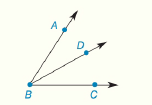Chapter 1.CR, Problem 20CR### Elementary Geometry for College St...

6th Edition
Daniel C. Alexander + 1 other
ISBN: 9781285195698

#### Solutions

Chapter
Section### Elementary Geometry for College St...

6th Edition
Daniel C. Alexander + 1 other
ISBN: 9781285195698
Textbook Problem
23 views

# Given: B D → bisects ∠ A B C m ∠ A B D = 2 x + 15 m ∠ D B C = 3 x + 5 Find: m ∠ A B CTo determine

To find:

The value of mABC.

Explanation

Given:

mABD=2x+15

mDBC=3x+5

And,

BD bisects ABC.

The Figure (1) is given as,

Figure (1)

Approach:

The bisector of an angle is the ray that separates the given angle into two congruent angles.

Calculation:

Since, BD is bisecting the angle ABC then it can be written as,

mABD=mDBC(1)

mABC=2×mABD(2)

Substitute 2x+15 for mABD and 3x+5 for mDBC in the above equation (1).

### Still sussing out bartleby?

Check out a sample textbook solution.

See a sample solution

#### The Solution to Your Study Problems

Bartleby provides explanations to thousands of textbook problems written by our experts, many with advanced degrees!

Get Started

#### In Exercises 13-20, sketch a set of coordinate axes and plot each point. 15. (3, 1)

Applied Calculus for the Managerial, Life, and Social Sciences: A Brief Approach

#### True or False: is a convergent series.

Study Guide for Stewart's Single Variable Calculus: Early Transcendentals, 8th

#### Define a construct and explain the role that constructs play in theories.

Research Methods for the Behavioral Sciences (MindTap Course List)

#### Graph each hyperbola. xy=20

College Algebra (MindTap Course List)